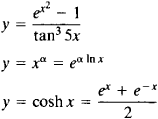# Elementary Function

(redirected from Elementary functions)
Also found in: Acronyms.

## elementary function

[‚el·ə′men·trē ′fəŋk·shən]
(mathematics)
Any function which can be formed from algebraic functions and the exponential, logarithmic, and trigonometric functions by a finite number of operations consisting of addition, subtraction, multiplication, division, and composition of functions.
McGraw-Hill Dictionary of Scientific & Technical Terms, 6E, Copyright © 2003 by The McGraw-Hill Companies, Inc.
The following article is from The Great Soviet Encyclopedia (1979). It might be outdated or ideologically biased.

## Elementary Function

any function in the class consisting of polynomials, rational functions, exponential functions, logarithmic functions, trigonometric functions, and inverse trigonometric functions. The class of elementary functions also includes functions obtained from those listed above through the performance of the four arithmetic operations and composition (the formation of a composite function) a finite number of times; examples areThe class of elementary functions has been best studied, and is most often encountered, in applications of mathematics. Many practical problems, however, lead to the consideration of functions that are not elementary functions, such as cylindrical functions. The derivative of an elementary function is also an elementary function. The indefinite integral of an elementary function is not always expressed in terms of an elementary function. Nonelementary functions are often represented in terms of elementary functions through the use of, for example, infinite series, infinite products, and infinite integrals.

References in periodicals archive ?
For each elementary function, we searched for at least one solution based on a literature review, analysis of similar systems in the market or patented systems, and application of the brainstorming method as proposed by BACK et al.
In [1,5], the authors derived new uniform convergent expansions of the incomplete gamma function [gamma](a, z) and the Bessel functions [J.sub.v](z) and [Y.sub.v](z) in terms of elementary functions of z that hold uniformly in unbounded regions of C containing the point z = 0.
The potential is in general given parametrically; however, in several cases the involved coordinate transformation allows inversion thus leading to particular potentials which are explicitly written in terms of elementary functions. These reductions are achieved by particular specifications of a parameter standing for the third finite singularity of the general Heun equation.
Now we shall call the function defined in (1) [mathematical expression not reproducible] since it does not refer to anyone and it has unknown analytic representation as elementary function using standard special functions and the RHS of (18) presents another representation of T(x) function using CDF of the normal distribution.
Therefore, one major consequence should be that the RTD function of this zone can theoretically be calculated by a proper but simple combination of the elementary function of the EZ or KN elements at the same feed rate and speed.
We have shown, with the help of a conformally flat metric, that these integrals maybe evaluated in terms of elementary functions. This example suggests that our approach may be extended to other physically relevant metrics.
Representing an image with a few elementary functions is widely used in image processing and computer vision.
It is obvious that the term [MATHEMATICAL EXPRESSION NOT REPRODUCIBLE IN ASCII] ds can not solved directly due to [MATHEMATICAL EXPRESSION NOT REPRODUCIBLE IN ASCII] ds can not be expressed by elementary functions. But, applying Theorem 3.3 we can give an upper bound 10/3 [e.sup.2]+ [[square root of [e.sup.3]]/3] for (17).
In some way or another, each looks at elementary functions from the perspective of experimental mathematics.
We see from Examples 1 to 4 that, in general, for most elementary functions (such as polynomials, rational functions, radical functions, and trigonometric functions) we can use algebraic identities to figure out their respective derivatives (the largest constant) of respective functions; although sometimes we need to elaborate on finding proper inequalities.
It is easy to compute for usual elementary functions. When no efficient algorithm exists for the computation of [f], it can be approximated by an inclusion function F satisfying the following definition.
where the integral for f(x) cannot be written in terms of elementary functions. A use of the definite integral is to determine the area between a curve and the horizontal axis (see Figure 1).

Site: Follow: Share:
Open / Close